Students can download Maths Chapter 4 Geometry Ex 4.4 Questions and Answers, Notes, Samacheer Kalvi 10th Maths Guide Pdf helps you to revise the complete Tamilnadu State Board New Syllabus, helps students complete homework assignments and to score high marks in board exams.

## Tamilnadu Samacheer Kalvi 10th Maths Solutions Chapter 4 Geometry Ex 4.4

Question 1.
The length of the tangent to a circle from a point P, which is 25 cm away from the centre is 24 cm. What is the radius of the circle?
Solution:
Let the radius AB be r. In the right ∆ ABO,
OB2 = OA2 + AB2
252 = 242 + r2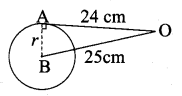252 – 242 = r2
(25 + 24) (25 – 24) = r2
r = $$\sqrt { 49 }$$ =7
Radius of the circle = 7 cmQuestion 2.
∆ LMN is a right angled triangle with ∠L = 90°. A circle is inscribed in it. The lengths of the sides containing the right angle are 6 cm and 8 cm. Find the radius of the circle.
Solution:
LN = 6; ML = 8. In the right ∆ LMN,
MN2 = LN2 + LM2
= 62 + 82 = 36 + 64 = 100
MN = $$\sqrt { 100 }$$ = 10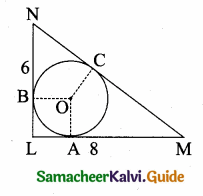OA= OB = OC = r
AN = CN (Tangent of the circle)
LN – AL= CN
LN – r = CN
8 – r = CN ……(1)
MC = MB (tangent of the circle)
MC = ML – LB
MC = 6 – r …….(2)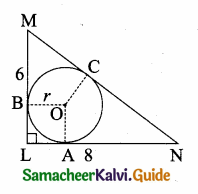MC + CN = (6 – r) + (8 – r)
MN = 14 – 2r
10 = 14 – 2r
2r = 14 – 10 = 4
r = $$\frac { 4 }{ 2 }$$ = 2 cm
radius of the circle = 2 cmQuestion 3.
A circle is inscribed in ∆ ABC having sides 8 cm, 10 cm and 12 cm as shown in figure, Find AD, BE and CF.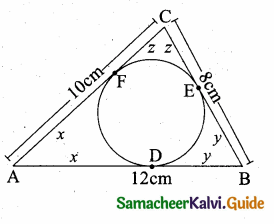Solution:
AD = AF = x (tangent of the circle)
BD = BE = y (tangent of the circle)
CE = CF = z (tangent of the circle)
x + y = 12 ……(1)
BC = BE + EC
y + z= 8 …….(2)
AC = AF + FC
x + z = 10 ……(3)
2x + 2y + 2z = 12 + 8 + 10
x + y + z = $$\frac { 30 }{ 2 }$$ = 15 …….(4)
By x + y = 12 in (4)
z = 3
y + z = 8 in (4)
x = 7
x + z = 10 in (4)
y = 5
AD = 7 cm; BE = 5 cm and CF = 3 cmQuestion 4.
PQ is a tangent drawn from a point P to a circle with centre O and QOR is a diameter of the circle such that ∠POR = 120° . Find ∠OPQ.
Solution:
∠POQ = 180° – 120° = 60°
In ∆OPQ, we know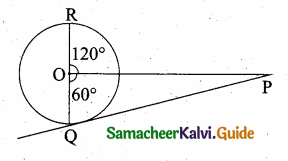∠POQ + ∠OQP + ∠OPQ = 180°
(Sum of the angles of a ∆ is 180°)
60° + 90° + ∠OPQ = 180°
∠OPQ = 180° – 150° = 30°
∠OPQ = 30°Question 5.
A tangent ST to a circle touches it at B. AB is a chord such that ∠ABT = 65°. Find ∠AOB, where “O” is the centre of the circle.
Solution:
Given ∠ABT = 65°
∠OBT = 90°(TB is the tangent of the circle)
∠ABO = 90° – 65° = 25°
∠ABO + ∠BOA + ∠OAB = 180°
25° + x + 25° = 180° (Sum of the angles of a ∆)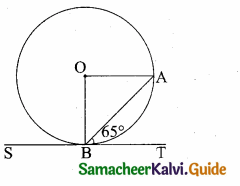(OA and OB are the radius of the circle.
∴ ∠ABO = ∠BAO = 25°
x + 50 = 180°
x = 180° – 50° = 130°
∴ ∠BOA = 130°

Question 6.
In figure, O is the centre of the circle with radius 5 cm. T is a point such that OT = 13 cm and OT intersects the circle E, if AB is the tangent to the circle at E, find the length of AB.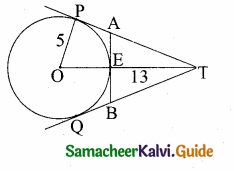Solution:
In the right ∆ OTP,
PT2 = OT2 – OP2
= 132 – 52
= 169 – 25
= 144
PT = $$\sqrt { 144 }$$ = 12 cm
Since lengths of tangent drawn from a point to circle are equal.
∴ AP = AE = x
AT = PT – AP
= (12 – x) cm
Since AB is the tangent to the circle E.
∴ OE ⊥ AB
∠OEA = 90°
∠AET = 90°
In ∆AET, AT2 = AE2 + ET2
In the right triangle AET,
AT2 = AE2 + ET2
(12 – x)2 = x2 + (13 – 5)2
144 – 24x + x2 = x2 + 64
24x = 80 ⇒ x = $$\frac { 80 }{ 24 }$$ = $$\frac { 20 }{ 6 }$$ = $$\frac { 10 }{ 3 }$$
BE = $$\frac { 10 }{ 3 }$$ cm
AB = AE + BE
= $$\frac { 10 }{ 3 }$$ + $$\frac { 10 }{ 3 }$$ = $$\frac { 20 }{ 3 }$$
Lenght of AB = $$\frac { 20 }{ 3 }$$ cmQuestion 7.
In two concentric circles, a chord of length 16 cm of larger circle becomes a tangent to the smaller circle whose radius is 6 cm. Find the radius of the larger circle.
Solution:
Here AP = PB = 8 cm
In ∆OPA,
OA2 = OP2 + AP2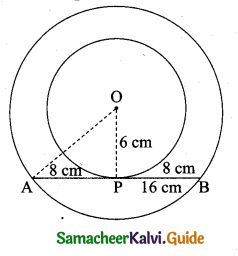= 62 + 82
= 36 + 64
= 100
OA = $$\sqrt { 100 }$$ = 10 cm
Radius of the larger circle = 10 cmQuestion 8.
Two circles with centres O and O’ of radii 3 cm and 4 cm, respectively intersect at two points P and Q, such that OP and O’ P are tangents to the two circles. Find the length of the common chord PQ.
Solution:
In ∆ OO’P
(O’O)2 = OP2 + O’P2
= 32 + 42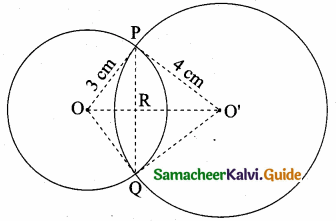= 9 + 16
(OO’)2 = 25
∴ OO’ = 5cm
Since the line joining the centres of two intersecting circles is perpendicular bisector of their common chord.
OR ⊥ PQ and PR = RQ
Let OR be x, then O’R = 5 – x again Let PR = RQ = y cm
In ∆ ORP, OP2 = OR2 + PR2
9 = x2 + y2 …(1)
In ∆ O’RP, O’P2 = O’R2 + PR2
16 = (5 – x)2 + y2
16 = 25 + x2 – 10x + y2
16 = x2 + y2 + 25 – 10x
16 = 9 + 25 – 10x (from 1)
16 = 34 – 10x
10x = 34 – 16 = 18
x = $$\frac { 18 }{ 10 }$$ = 1.8 cm
Substitute the value of x = 1.8 in (1)
9 = (1.8)2 + y2
y2 = 9 – 3.24
y2 = 5.76
y = $$\sqrt { 5.76 }$$ = 2.4 cm
Hence PQ = 2 (2.4) = 4.8 cm
Length of the common chord PQ = 4.8 cmQuestion 9.
Show that the angle bisectors of a triangle are concurrent.
Solution:
Given: ABC is a triangle. AD, BE and CF are the angle bisector of ∠A, ∠B, and ∠C.
To Prove: Bisector AD, BE and CF intersect
Proof: The angle bisectors AD and BE meet at O. Assume CF does not pass through O. By angle bisector theorem.
AD is the angle bisector of ∠A
$$\frac { BD }{ DC }$$ = $$\frac { AB }{ AC }$$ …..(1)
BE is the angle bisector of ∠B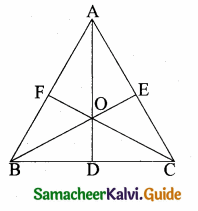$$\frac { CE }{ EA }$$ = $$\frac { BC }{ AB }$$ …….(2)
CF is the angle bisector ∠C
$$\frac { AF }{ FB }$$ = $$\frac { AC }{ BC }$$ …….(3)
Multiply (1) (2) and (3)
$$\frac { BD }{ DC }$$ × $$\frac { CE }{ EA }$$ × $$\frac { AF }{ FB }$$ = $$\frac { AB }{ AC }$$ × $$\frac { BC }{ AB }$$ × $$\frac { AC }{ BC }$$
So by Ceva’s theorem.
The bisector AD, BE and CF are concurrent.Question 10.
In ∆ABC , with ∠B = 90° , BC = 6 cm and AB = 8 cm, D is a point on AC such that AD = 2 cm and E is the midpoint of AB. Join D to E and extend it to meet at F. Find BF.
Solution:
Consider ∆ABC. Then D, E and F are respective points on the sides AC, AB and BC.
By construction D, E, F are collinear.
By Menelaus’ theorem $$\frac { AE }{ EB }$$ × $$\frac { BF }{ FC }$$ × $$\frac { CD }{ DA }$$ = 1 ……(1)
AD = 2 cm; AE = EB = 4 cm; BC = 6 cm; FC = FB + BC = x + 6
In ∆ABC, By Pythagoras theorem.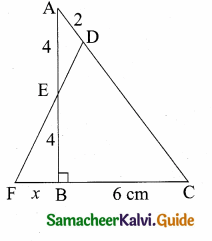AC2= AB2 + BC2
AC2 = 82 + 62 = 64 + 36 = 100
AC = $$\sqrt { 100 }$$ = 10
= 10 – 2 = 8 cm
Substituting the values in (1) we get
$$\frac { 4 }{ 4 }$$ × $$\frac { x }{ x+6 }$$ × $$\frac { 8 }{ 2 }$$ = 1
$$\frac { x }{ x+6 }$$ × 4 = 1
4x = x + 6
3x = 6 ⇒ x = $$\frac { 6 }{ 3 }$$ = 2
The value of BF = 2 cm

Question 11.
An artist has created a triangular stained glass window and has one strip of small length left before completing the window. She needs to figure out the length of left out portion based on the lengths of the other sides as shown in the figure.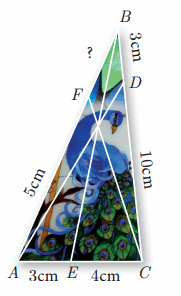Solution:
Given that AE = 3 cm, EC = 4 cm, CD = 10 cm, DB = 3 cm, AF = 5 cm.
Let FB be x
Using Ceva’s theorem we have
$$\frac { AE }{ EC }$$ × $$\frac { CD }{ DB }$$ × $$\frac { BF }{ AF }$$ = 1
$$\frac { 3 }{ 4 }$$ × $$\frac { 10 }{ 3 }$$ × $$\frac { x }{ 5 }$$ = 1
$$\frac { 2x }{ 4 }$$ = 1
2x = 4 ⇒ x = $$\frac { 4 }{ 2 }$$ = 2
The value of BF = 2Question 12.
Draw a tangent at any point R on the circle of radius 3.4 cm and centre at P ?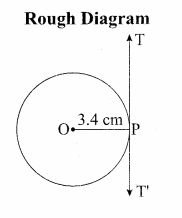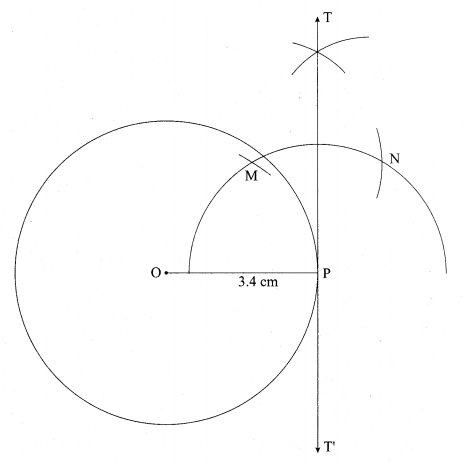Steps of construction:

1. Draw a circle with centre “O” of radius 3.4 cm.
2. Take a point P on the circle Join OP.
3. Draw a perpendicular line TT’ to OP which passes through P.
4. TT’ is the required tangent.Question 13.
Draw a circle of radius 4.5 cm. Take a point on the circle. Draw the tangent at that point using the alternate segment theorem.
Radius of the circle = 4.5 cm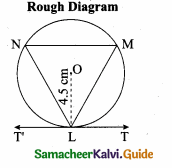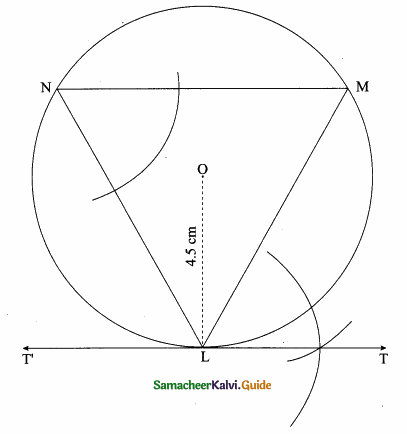Steps of construction:

1. With O as centre, draw a circle of radius 4.5 cm.
2. Take a point L on the circle. Through L draw any chord LM.
3. Take a point M distinct from L and N on the circle, so that L, M, N are in anti-clockwise direction. Join LN and NM.
4. Through “L” draw tangent TT’such that ∠TLM = ∠MNL
5. TT’ is the required tangent.Question 14.
Draw the two tangents from a point which is 10 cm away from the centre of a circle of radius 5 cm. Also, measure the lengths of the tangents.
Radius = 5 cm; Distance = 10 cm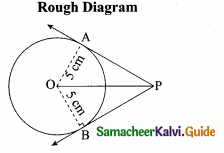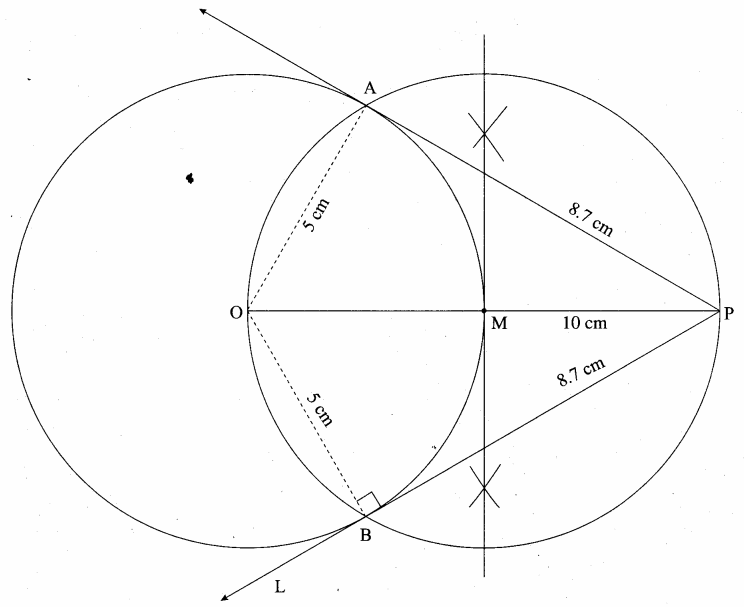Steps of construction:

1. With O as centre, draw a circle of radius 5 cm.
2. Draw a line OP =10 cm.
3. Draw a perpendicular bisector of OP, which cuts OP at M.
4. With M as centre and MO as radius draw a circle which cuts previous circle at A and B.
5. Join AP and BP. AP and BP are the required tangents.Verification: In the right ∆ OAP
PA2 = OP2 – OA2
= 102 – 52 = $$\sqrt { 100-25 }$$ = $$\sqrt { 75 }$$ = 8.7 cm
Lenght of the tangent is = 8.7 cm

Question 15.
Take a point which is 11 cm away from the centre of a circle of radius 4 cm and draw the two tangents to the circle from that point.
Radius = 4 cm; Distance = 11 cm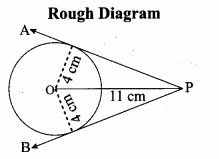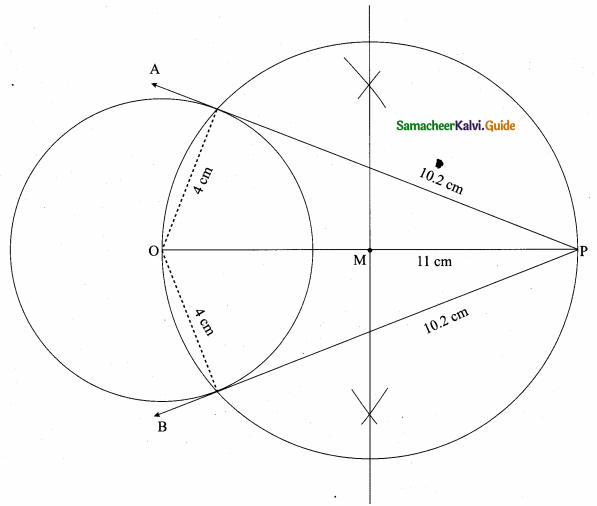Steps of construction:

1. With O as centre, draw a circle of radius 4 cm.
2. Draw a line OP = 11 cm.
3. Draw a perpendicular bisector of OP, which cuts OP at M.
4. With M as centre and MO as radius, draw a circle which cuts previous circle A and B.
5. Join AP and BP. AP and BP are the required tangents.

This the length of the tangents PA = PB = 10.2 cm
Verification: In the right angle triangle OAP
PA2 = OP2 – OA2
= 112 – 42 = 121 – 16 = 105
PA = $$\sqrt { 105 }$$ = 10.2 cm
Length of the tangents = 10.2 cmQuestion 16.
Draw the two tangents from a point which is 5 cm away from the centre of a circle of diameter 6 cm. Also, measure the lengths of the tangents.
Radius = 3cm; Distance = 5cm.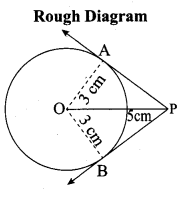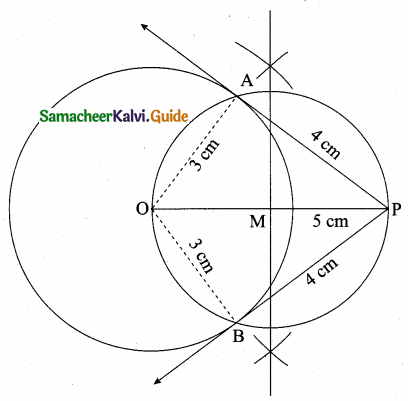Steps of construction:

1. With O as centre, draw a circle of radius 3 cm.
2. Draw a line OP = 5 cm.
3. Draw a perpendicular bisector of OP, which cuts OP at M.
4. With M as centre and MO as radius draw a circle which cuts previous circles at A and B.
5. Join AP and BP, AP and BP are the required tangents.

The length of the tangent PA = PB = 4 cm
Verification: In the right angle triangle OAP
PA2 = OP2 – OA2
= 52 – 32
= 25 – 9
= 16 PA
= $$\sqrt { 16 }$$ = 4 cm
Length of the tangent = 4 cmQuestion 17.
Draw a tangent to the circle from the point P having radius 3.6 cm, and centre at O. Point P is at a distance 7.2 cm from the centre.
Radius = 3.6; Distance = 7.2 cm.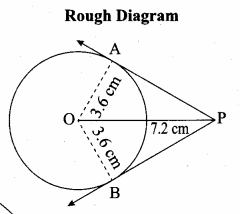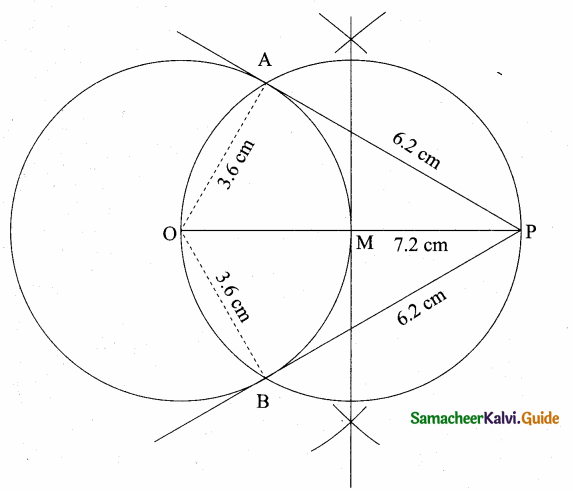Steps of construction:

1. With O as centre, draw a circle of radius 3.6 cm.
2. Draw a line OP = 7.2 cm.
3. Draw a perpendicular bisector of OP which cuts OP at M.
4. With M as centre and MO as radius draw a circle which cuts the previous circle at A and B.
5. Join AP and BP, AP and BP are the required tangents.Length of the tangents PA = PB = 6.26 cm
Verification: In the right triangle ∆OAP
PA2 = OP2 – OA2
= 7.22 – 3.62 =(7.2 + 3.6) (7.2 – 3.6)
PA2 = 10.8 × 3.6 = $$\sqrt { 38.88 }$$
PA = 6.2 cm
Length of the tangent = 6.2 cm